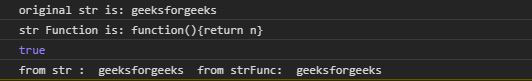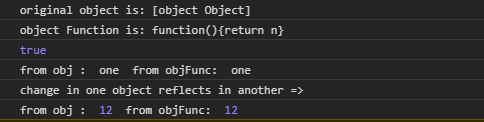Open in App
Not now

# Underscore.js _.constant() Function

• Last Updated : 10 Jul, 2020

Underscore.js is a JavaScript library that makes operations on arrays, string, objects much easier and handy. The _.constant() function is used to create a function that returns the parameter given to it. It is almost same as _.identity() function.

Note: It is very necessary to link the underscore CDN before going and using underscore functions in the browser. When linking the underscore.js CDN, the “_” is attached to the browser as global variable.

Syntax:

`_.constant( object );`

Parameters: This function accepts single parameter object.

Return Value: This function returns the parameter as given to the function.

Below examples illustrate the _.constant() function in Underscore.js:

Example 1:

 ` ` `<``html``> ` ` `  `<``head``> ` `    ``<``script` `src``= ` `"https://cdnjs.cloudflare.com/ajax/libs/underscore.js/1.9.1/underscore-min.js"``> ` `    `` ` ` ` ` `  `<``body``> ` `    ``<``script``> ` ` `  `        ``let str = "geeksforgeeks" ` ` `  `        ``// _.constant function of underscore.js ` `        ``let strFunc = _.constant(str); ` `        ``console.log(`original str is: \${str}`) ` `        ``console.log(`str Function is: \${strFunc}`) ` ` `  `        ``// This will return true ` `        ``console.log(str === strFunc()) ` ` `  `        ``// Both strings are exactly same ` `        ``console.log("from str : ", str,  ` `            ``" from strFunc: ", strFunc()); ` `    `` ` ` ` ` `  ` `

Output:Example 2:

 ` ` `<``html``> ` ` `  `<``head``> ` `    ``<``script` `src``= ` `"https://cdnjs.cloudflare.com/ajax/libs/underscore.js/1.9.1/underscore-min.js"``> ` `    `` ` ` ` ` `  `<``body``> ` `    ``<``script``> ` ` `  `        ``// Creating a object ` `        ``let obj = { ` `            ``"a": "one", ` `            ``"b": "two", ` `            ``"c": "three" ` `        ``} ` ` `  `        ``// _.constant function of underscore.js ` `        ``let objFunc = _.constant(obj); ` `        ``console.log(`original object is: \${obj}`) ` `        ``console.log(`object Function is: \${objFunc}`) ` ` `  `        ``// This will return true ` `        ``console.log(obj === objFunc()) ` ` `  `        ``// Both objects are exactly same ` `        ``console.log("from obj : ", obj.a,  ` `            ``" from objFunc: ", objFunc().a); ` ` `  `        ``// Made Changes in object ` `        ``obj.a = 12 ` ` `  `        ``// Change in one object reflects ` `        ``// in another ` `        ``console.log("change in one object " ` `                ``+ "reflects in another =>") ` `                 `  `        ``console.log("from obj : ", obj.a,  ` `            ``" from objFunc: ", objFunc().a); ` `    `` ` ` ` ` `  ` `

Output:My Personal Notes arrow_drop_up
Related Articles